Function Repository Resource:

# Nullity

Compute the nullity of a matrix

Contributed by: Wolfram|Alpha Math Team
 ResourceFunction["Nullity"][mat] computes the nullity of the matrix mat.

## Details and Options

The nullity of a matrix is the dimension of its null space, also called its kernel. The kernel is the space of all input vectors that the matrix maps to zero.

## Examples

### Basic Examples (3)

Compute the nullity of a matrix:

 In:=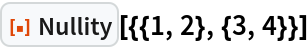Out=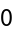Compute the nullity of another matrix:

 In:=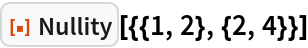Out=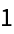Compute the nullity of another matrix:

 In:=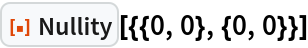Out=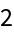### Properties and Relations (5)

The rank-nullity theorem states that the rank of a matrix plus its nullity equals its column count:

 In:=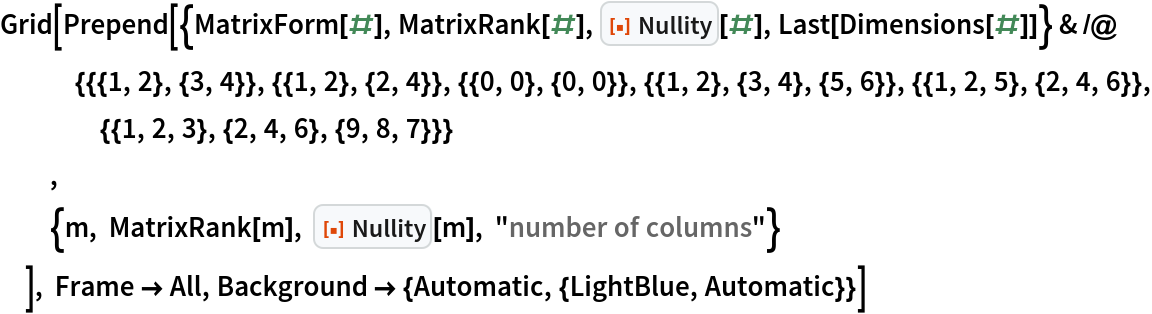Out=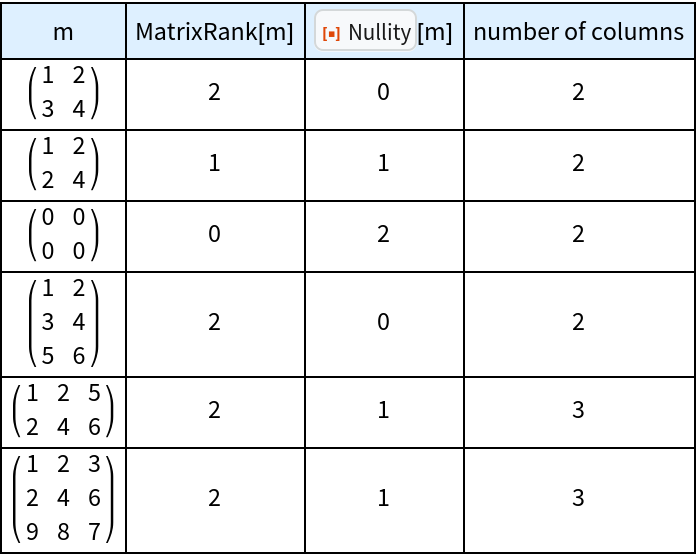When called with symbolic arguments, Nullity assumes maximal linear independence:

 In:=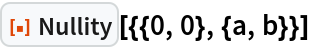Out=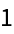The nullity of an identity matrix is always zero:

 In:=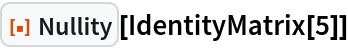Out=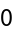The nullity of a Hilbert matrix is always zero:

 In:=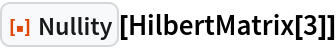Out=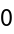The nullity of a Toeplitz matrix is always zero:

 In:=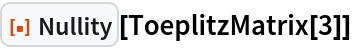Out=## Publisher

Wolfram|Alpha Math Team

## Version History

• 3.0.0 – 23 March 2023
• 2.1.0 – 12 May 2021
• 2.0.0 – 24 January 2020
• 1.0.0 – 17 September 2019